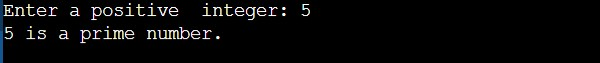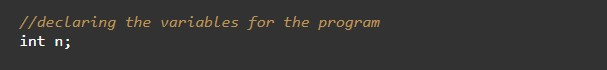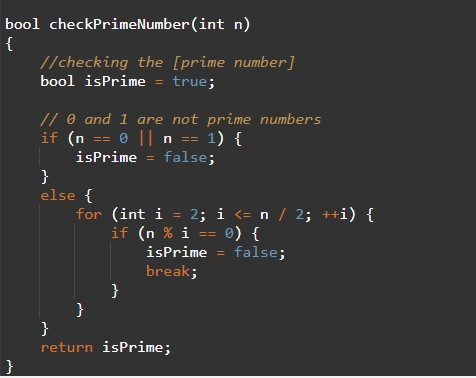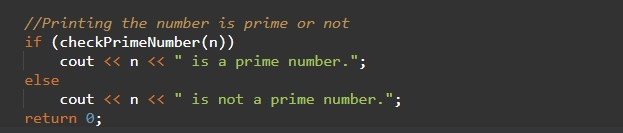# C++ Program to Check Prime Number Using Function

In this tutorial you will learn about the C++ Program to Check Prime Number Using Function and its application with practical example.

## C++ Program to Check Prime Number Using Function

In this tutorial, we will learn to create a C++ program that will Check Prime Number Using functions using C++ programming.

## Prerequisites

Before starting with this tutorial we assume that you are best aware of the following C++ programming topics:

• Operators in C++ Programming.
• Basic Input and Output function in C++ Programming.
• Basic C++ programming.
• For loop in C++ programming.
• Conditional Statements in C++ programming.
• Arithmetic operations in C++ Programming.

## Program to Check Prime Number Using Function:-

A prime number is a number that is only divisible by only one number or by itself only. In c++ programming, it is possible to take numerical input from the user and check if that number is Prime Number or not with the help of a very small amount of code.

In this program, we will take a number in the input from the user to check if it’s prime or not.

With the help of this program, we can Check Prime Number Using Function.

## Output:-In the above program, we have first initialized the required variable.• no= it will hold the integer value of the input.
• i = it will hold the integer value.
• flag = it will hold the integer value.

Input message for the user for the integer value.Program Logic Code.Printing output prime number or not.In this tutorial we have learn about the C++ Program to Check Prime Number Using Function and its application with practical example. I hope you will like this tutorial.